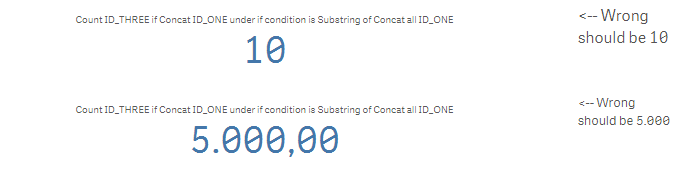# New to Qlik Sense

Discussion board where members can get started with Qlik Sense.

Announcements
Make your voice heard! Participate in the 2020 Wisdom of Crowds® Survey. BEGIN SURVEYNot applicable

## Sum selected values using concat and if

ID_ONE ID_TWO ID_THREE IA 1 10 100 1000 2 11 101 2000 2 11 102 3000 2 12 103 4000 3 13 104 5000 4 14 105 6000 4 15 106 7000

Hello Dear Community,

I am looking for a way to sum the complete IA of ID_One if ID_ONE is present in one row selected by if conditions.

So first I want to selct the ID_ONE´s, which meet the If-conditions.

Then I want to sum the IA in all rows, in which ID_ONE is present.

Example:

Distinct If ((ID_TWO=11 or ID_TWO=12 or ID_TWO=13 or ID_TWO=14)

and (ID_THREE = 101 or  ID_THREE = 103 or ID_THREE = 105) ,ID_ONE)

This way I would get the ID_ONE´s  2 and 4.

And then I want to sum the belonging IA. So the result should be ((2000+3000+4000)+(6000+7000)) = 22.000.

To achieve this I thought of using Concat-function in combination with SubstringCount.

I have provided an Example App below. The data is a bit different. I tried it like this:

IF(SubstringCount(

Concat( Distinct ID_ONE,';'),

Concat(Distinct If([K_ONE]>2000 AND ([K_TWO]<2000 OR [K_Three]<>6) ,ID_ONE),';')

)=0,

Sum(IA))

I would be grateful if someone could help me with that.

Btw: I am using qlik Sense and the Limits for the if comparisions (11,12,13,14,2000, 6...) are actually Variables in my real Applikation.

Best regards

Tags (4)
1 Solution

Accepted Solutions
HighlightedNot applicable

## Re: Sum selected values using concat and if

Ok. The formula I found (actually a colleague, who works on the same Project) works perfectly fine and I think it is pretty amazing:

Sum(

If(

Substringcount(

Concat(ALL Distinct If(  (condition1) AND (condition2 OR condition3),ID_ONE),';'),Text(ID_ONE)

) >0,

IA)

)

With the Concat-function the set of ID_ONE´s, which meet the conditions, is made explicit in a String. In the next step this String is used via SubstringCount to select the IA I want to sum.

I think this method is a good alternative to Set Analysis.

Because in my opinion if-conditions are a lot easier to understand and to write than set Analysis expressions and you can easily make the set you are using visable.

Nevertheless I would guess the Performance of Set Analysis is better.

6 Replies
Highlighted
Valued Contributor

## Re: Sum selected values using concat and if

Hi

Why don;t you, go by like this ?

sum(      { \$<ID_ONE={"=COUNT(ID_ONE)>1"}>}    IA)

 This way I would get the ID_ONE´s  2 and 4. And then I want to sum the belonging IA. So the result should be ((2000+3000+4000)+(6000+7000)) = 22.000.
HighlightedNot applicable

## Re: Sum selected values using concat and if

Sure for this Little example that would work, but in the real App I have a lot more different fields and about 20 conditions. Just saying sum  where Count(ID_ONE)>1 would not work.Highlighted
Valued Contributor

## Re: Sum selected values using concat and if

Hi,

You could use set analysis for that, here is an example:

sum({\$<ID_TWO={11,12,13,14}>*\$<ID_THREE={101,103,105}>} total<ID_ONE> IA)

The expression above calculates the sum of IA aggregated by ID_ONE for the values where there is an intersection ( * ) between the first set \$<ID_TWO={11,12,13,14}> (same as your logic: ID_TWO=11 or ID_TWO=12 or ID_TWO=13 or ID_TWO=14) and the second set \$<ID_THREE={101,103,105}> (same as your logic: ID_THREE = 101 or  ID_THREE = 103 or ID_THREE = 105).

Let me know if that works.

Kind Regards,

Cesar

HighlightedNot applicable

## Re: Sum selected values using concat and if

I probably just found the solution. I am going to post it, if I can confirm it.

HighlightedMVP

## Re: Sum selected values using concat and if

Try this for count

=Count({<ID_ONE = P({<ID_TWO = {11, 12, 13, 14}>})+P({<ID_THREE = {101, 103, 105}>})>}ID_ONE)

and this for Sum

=Sum({<ID_ONE = P({<ID_TWO = {11, 12, 13, 14}>})+P({<ID_THREE = {101, 103, 105}>})>}IA)HighlightedNot applicable

## Re: Sum selected values using concat and if

Ok. The formula I found (actually a colleague, who works on the same Project) works perfectly fine and I think it is pretty amazing:

Sum(

If(

Substringcount(

Concat(ALL Distinct If(  (condition1) AND (condition2 OR condition3),ID_ONE),';'),Text(ID_ONE)

) >0,

IA)

)

With the Concat-function the set of ID_ONE´s, which meet the conditions, is made explicit in a String. In the next step this String is used via SubstringCount to select the IA I want to sum.

I think this method is a good alternative to Set Analysis.

Because in my opinion if-conditions are a lot easier to understand and to write than set Analysis expressions and you can easily make the set you are using visable.

Nevertheless I would guess the Performance of Set Analysis is better.